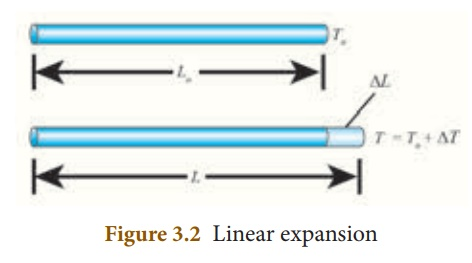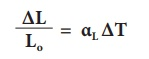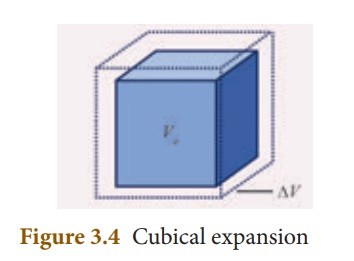Home | | Science 10th Std | Effect of Heat Energy

# Effect of Heat Energy

When a certain amount of heat energy is given to a substance, it will undergo one or more of the following changes: · Temperature of the substance rises. · The substance may change its state from solid to liquid or from liquid to gas. · The substance will expand when heated.

EFFECT OF HEAT ENERGY

When a certain amount of heat energy is given to a substance, it will undergo one or more of the following changes:

·                 Temperature of the substance rises.

·                 The substance may change its state from solid to liquid or from liquid to gas.

·                 The substance will expand when heated.

The rise in temperature is in proportion to the amount of heat energy supplied. It also depends on the nature and mass of the substance. About the rise in temperature and the change of state, you have studied in previous classes. In the following section, we shall discuss about the expansion of substances due to heat.

## 1. Expansion of Substances

When heat energy is supplied to a body, there can be an increase in the dimension of the object. This change in the dimension due to rise in temperature is called thermal expansion of the object. The expansion of liquids (e.g. mercury) can be seen when a thermometer is placed in warm water. All forms of matter (solid, liquid and gas) undergo expansion on heating.

### a) Expansion in solidsWhen a solid is heated, the atoms gain energy and vibrate more vigorously. This results in the expansion of the solid. For a given change in temperature, the extent of expansion is smaller in solids than in liquids and gases. This is due to the rigid nature of solids.

The different types of expansion of solid are listed and explained below:

1.              Linear expansion

2.              Superficial expansion

3.              Cubical expansion

1.              Linear expansion:

When a body is heated or cooled, the length of the body changes due to change in its temperature. Then the expansion is said to be linear or longitudinal expansion.

The ratio of increase in length of the body per degree rise in temperature to its unit length is called as the coefficient of linear expansion. The SI unit of Coefficient of Linear expansion is K -1. The value of coefficient of linear expansion is different for different materials.The equation relating the change in length and the change in temperature of a body is given below:∆ L -  Change in length (Final length-Original length)

Lo - Original length

∆T -  Change in temperature (Final temperature - Initial temperature)

αL - Coefficient of linear expansion.

### 2. Superficial expansion:

If there is an increase in the area of a solid object due to heating, then the expansion is called superficial or areal expansion.

Superficial expansion is determined in terms of coefficient of superficial expansion. The ratio of increase in area of the body per degree rise in temperature to its unit area is called as coefficient of superficial expansion. Coefficient of superficial expansion is different for different materials. The SI unit of Coefficient of superficial expansion is K-1

The equation relating to the changein area and the change in temperature is given below:∆A -  Change in area (Final area - Initial area)

Ao - Original area

∆T -  Change in temperature (Final temperature - Initial temperature)

αA - Coefficient of superficial expansion.

### 3. Cubical expansion:

If there is an increase in the volume of a solid body due to heating, then the expansion is called cubical or volumetric expansion.

As in the cases of linear and areal expansion, cubical expansion is also expressed in terms of coefficient of cubical expansion. The ratio of increase in volume of the body per degree rise in temperature to its unit volume is called as coefficient of cubical expansion. This is also measured in K–1.The equation relating to the change in volume and the change in temperature is given below:∆V -  Change in volume(Final volume - Intial volume)

Vo - Original volume

∆T -  Change in temperature (Final temperature - Initial temperature)

αV - Coefficient of cubical expansion.

Different materials possess different coefficient of cubical expansion. Table 3.1 gives the coefficient of cubical expansion for some common materials.### b) Expansion in liquids and gases

When heated, the atoms in a liquid or gas gain energy and are forced further apart. The extent of expansion varies from substance to substance. For a given rise in temperature, a liquid will have more expansion than a solid and a gaseous substance has the highest expansion when compared with the other two. The coefficient of cubical expansion of liquid is independent of temperature whereas its value for gases depends on the temperature of gases.

When a liquid is heated, it is done by keeping the liquid in some container and supplying heat energy to the liquid through the container. The thermal energy supplied will be partly used in expanding the container and partly used in expanding the liquid. Thus, what we observe may not be the actual or real expansion of the liquid. Hence, for liquids, we can define real expansion and apparent expansion.

### 1) Real expansion

If a liquid is heated directly without using any container, then the expansion that you observe is termed as real expansion of the liquid.

Coefficient of real expansion is defined as the ratio of the true rise in the volume of the liquid per degree rise in temperature to its unit volume. The SI unit of coefficient of real expansion is K–1.

### 2) Apparent expansion

Heating a liquid without using a container is not possible. Thus, in practice, you can heat any liquid by pouring it in a container. A part of thermal energy is used in expanding the container and a part is used in expanding the liquid. Thus, what you observe is not the actual or real expansion of the liquid. The expansion of a liquid apparently observed without considering the expansion of the container is called the apparent expansion of the liquid.

Coefficient of apparent expansion is defined as the ratio of the apparent rise in the volume of the liquid per degree rise in temperature to its unit volume. The SI unit of coefficient of apparent expansion is K–1.

## 2. Experiment to measure realand apparent expansion of liquid

To start with, the liquid whose real and apparent expansion is to be determined is poured in a container up to a level. Mark this level as L1. Now, heat the container and the liquid using a burner as shown in the Figure 3.5.

Initially, the container receives the thermal energy and it expands. As a result, the volume of the liquid appears to have reduced. Mark this reduced level of liquid as L2.

On further heating, the thermal energy supplied to the liquid through the container results in the expansion of the liquid. Hence, the level of liquid rises to L3. Now, the difference between the levels L1 and L3 is called as apparent expansion, and the difference between the levels L2 and L3 is called real expansion. The real expansion is always more than that of apparent expansion.Real expansion = L3 – L2

Apparent expansion = L3 – L1

Study Material, Lecturing Notes, Assignment, Reference, Wiki description explanation, brief detail
10th Science : Chapter 3 : Thermal Physics : Effect of Heat Energy |